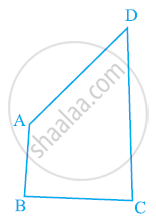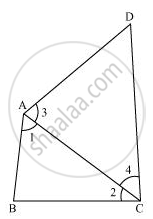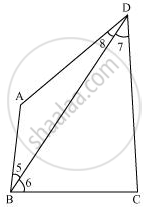# AB and CD are respectively the smallest and longest sides of a quadrilateral ABCD (see the given figure). Show that ∠A > ∠C and ∠B > ∠D. - Mathematics

AB and CD are respectively the smallest and longest sides of a quadrilateral ABCD (see the given figure). Show that ∠A > ∠C and ∠B > ∠D.#### SolutionLet us join AC.

In ΔABC,

AB < BC (AB is the smallest side of quadrilateral ABCD)

∴ ∠2 < ∠1 (Angle opposite to the smaller side is smaller) ... (1)

∴ ∠4 < ∠3 (Angle opposite to the smaller side is smaller) ... (2)

On adding equations (1) and (2), we obtain

∠2 + ∠4 < ∠1 + ∠3

⇒ ∠C < ∠A

⇒ ∠A > ∠C

Let us join BD.In ΔABD,

∴ ∠8 < ∠5 (Angle opposite to the smaller side is smaller) ... (3)

In ΔBDC,

BC < CD (CD is the largest side of quadrilateral ABCD)

∴ ∠7 < ∠6 (Angle opposite to the smaller side is smaller) ... (4)

On adding equations (3) and (4), we obtain

∠8 + ∠7 < ∠5 + ∠6

⇒ ∠D < ∠B

⇒ ∠B > ∠D

Concept: Inequalities in a Triangle
Is there an error in this question or solution?
Chapter 7: Triangles - Exercise 7.4 [Page 132]

#### APPEARS IN

NCERT Class 9 Maths
Chapter 7 Triangles
Exercise 7.4 | Q 4 | Page 132

Share